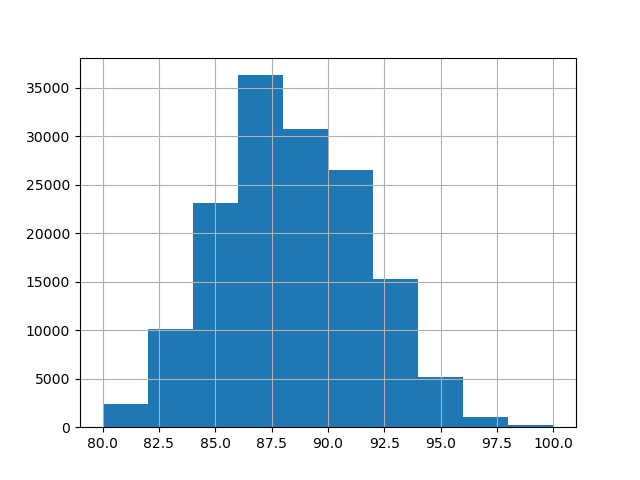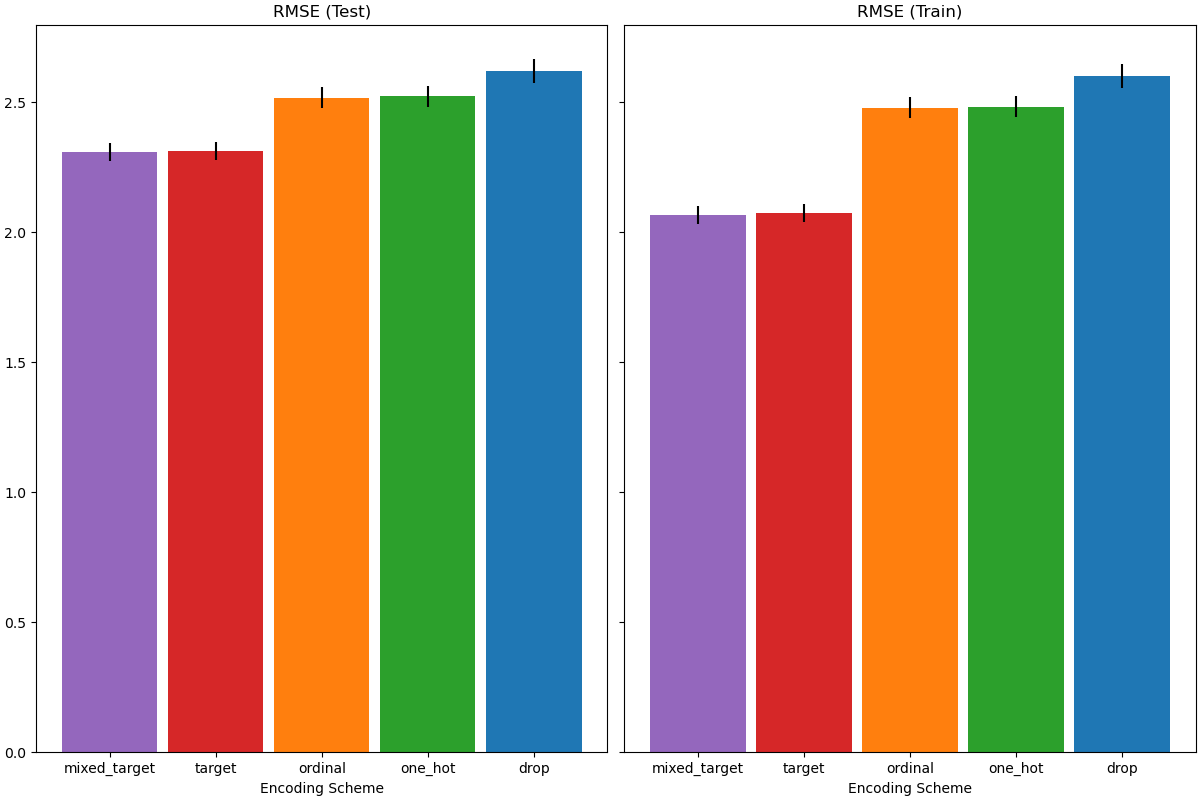# Comparing Target Encoder with Other Encoders¶

The TargetEncoder uses the value of the target to encode each categorical feature. In this example, we will compare three different approaches for handling categorical features: TargetEncoder, OrdinalEncoder, OneHotEncoder and dropping the category.

Note

fit(X, y).transform(X) does not equal fit_transform(X, y) because a cross fitting scheme is used in fit_transform for encoding. See the User Guide. for details.

First, we load the wine reviews dataset, where the target is the points given be a reviewer:

from sklearn.datasets import fetch_openml

wine_reviews = fetch_openml(data_id=42074, as_frame=True, parser="pandas")

df = wine_reviews.frame

country description designation points price province region_1 region_2 variety winery
0 US This tremendous 100% varietal wine hails from ... Martha's Vineyard 96 235.0 California Napa Valley Napa Cabernet Sauvignon Heitz
1 Spain Ripe aromas of fig, blackberry and cassis are ... Carodorum Selección Especial Reserva 96 110.0 Northern Spain Toro NaN Tinta de Toro Bodega Carmen Rodríguez
2 US Mac Watson honors the memory of a wine once ma... Special Selected Late Harvest 96 90.0 California Knights Valley Sonoma Sauvignon Blanc Macauley
3 US This spent 20 months in 30% new French oak, an... Reserve 96 65.0 Oregon Willamette Valley Willamette Valley Pinot Noir Ponzi
4 France This is the top wine from La Bégude, named aft... La Brûlade 95 66.0 Provence Bandol NaN Provence red blend Domaine de la Bégude

For this example, we use the following subset of numerical and categorical features in the data. The target are continuous values from 80 to 100:

numerical_features = ["price"]
categorical_features = [
"country",
"province",
"region_1",
"region_2",
"variety",
"winery",
]
target_name = "points"

X = df[numerical_features + categorical_features]
y = df[target_name]

_ = y.hist()## Training and Evaluating Pipelines with Different Encoders¶

In this section, we will evaluate pipelines with HistGradientBoostingRegressor with different encoding strategies. First, we list out the encoders we will be using to preprocess the categorical features:

from sklearn.compose import ColumnTransformer
from sklearn.preprocessing import OneHotEncoder, OrdinalEncoder, TargetEncoder

categorical_preprocessors = [
("drop", "drop"),
("ordinal", OrdinalEncoder(handle_unknown="use_encoded_value", unknown_value=-1)),
(
"one_hot",
OneHotEncoder(handle_unknown="ignore", max_categories=20, sparse_output=False),
),
("target", TargetEncoder(target_type="continuous")),
]


Next, we evaluate the models using cross validation and record the results:

from sklearn.ensemble import HistGradientBoostingRegressor
from sklearn.model_selection import cross_validate
from sklearn.pipeline import make_pipeline

n_cv_folds = 3
max_iter = 20
results = []

def evaluate_model_and_store(name, pipe):
result = cross_validate(
pipe,
X,
y,
scoring="neg_root_mean_squared_error",
cv=n_cv_folds,
return_train_score=True,
)
rmse_test_score = -result["test_score"]
rmse_train_score = -result["train_score"]
results.append(
{
"preprocessor": name,
"rmse_test_mean": rmse_test_score.mean(),
"rmse_test_std": rmse_train_score.std(),
"rmse_train_mean": rmse_train_score.mean(),
"rmse_train_std": rmse_train_score.std(),
}
)

for name, categorical_preprocessor in categorical_preprocessors:
preprocessor = ColumnTransformer(
[
("numerical", "passthrough", numerical_features),
("categorical", categorical_preprocessor, categorical_features),
]
)
pipe = make_pipeline(
)
evaluate_model_and_store(name, pipe)


## Native Categorical Feature Support¶

In this section, we build and evaluate a pipeline that uses native categorical feature support in HistGradientBoostingRegressor, which only supports up to 255 unique categories. In our dataset, the most of the categorical features have more than 255 unique categories:

n_unique_categories = df[categorical_features].nunique().sort_values(ascending=False)
n_unique_categories

winery      14810
region_1     1236
variety       632
province      455
country        48
region_2       18
dtype: int64


To workaround the limitation above, we group the categorical features into low cardinality and high cardinality features. The high cardinality features will be target encoded and the low cardinality features will use the native categorical feature in gradient boosting.

high_cardinality_features = n_unique_categories[n_unique_categories > 255].index
low_cardinality_features = n_unique_categories[n_unique_categories <= 255].index
mixed_encoded_preprocessor = ColumnTransformer(
[
("numerical", "passthrough", numerical_features),
(
"high_cardinality",
TargetEncoder(target_type="continuous"),
high_cardinality_features,
),
(
"low_cardinality",
OrdinalEncoder(handle_unknown="use_encoded_value", unknown_value=-1),
low_cardinality_features,
),
],
verbose_feature_names_out=False,
)

# The output of the of the preprocessor must be set to pandas so the
# gradient boosting model can detect the low cardinality features.
mixed_encoded_preprocessor.set_output(transform="pandas")
mixed_pipe = make_pipeline(
mixed_encoded_preprocessor,
random_state=0, max_iter=max_iter, categorical_features=low_cardinality_features
),
)
mixed_pipe

Pipeline(steps=[('columntransformer',
ColumnTransformer(transformers=[('numerical', 'passthrough',
['price']),
('high_cardinality',
TargetEncoder(target_type='continuous'),
Index(['winery', 'region_1', 'variety', 'province'], dtype='object')),
('low_cardinality',
OrdinalEncoder(handle_unknown='use_encoded_value',
unknown_value=-1),
Index(['country', 'region_2'], dtype='object'))],
verbose_feature_names_out=False)),
max_iter=20, random_state=0))])
In a Jupyter environment, please rerun this cell to show the HTML representation or trust the notebook.

Finally, we evaluate the pipeline using cross validation and record the results:

evaluate_model_and_store("mixed_target", mixed_pipe)


## Plotting the Results¶

In this section, we display the results by plotting the test and train scores:

import matplotlib.pyplot as plt
import pandas as pd

results_df = (
pd.DataFrame(results).set_index("preprocessor").sort_values("rmse_test_mean")
)

fig, (ax1, ax2) = plt.subplots(
1, 2, figsize=(12, 8), sharey=True, constrained_layout=True
)
xticks = range(len(results_df))
name_to_color = dict(
zip((r["preprocessor"] for r in results), ["C0", "C1", "C2", "C3", "C4"])
)

for subset, ax in zip(["test", "train"], [ax1, ax2]):
mean, std = f"rmse_{subset}_mean", f"rmse_{subset}_std"
data = results_df[[mean, std]].sort_values(mean)
ax.bar(
x=xticks,
height=data[mean],
yerr=data[std],
width=0.9,
color=[name_to_color[name] for name in data.index],
)
ax.set(
title=f"RMSE ({subset.title()})",
xlabel="Encoding Scheme",
xticks=xticks,
xticklabels=data.index,
)When evaluating the predictive performance on the test set, dropping the categories perform the worst and the target encoders performs the best. This can be explained as follows:

• Dropping the categorical features makes the pipeline less expressive and underfitting as a result;

• Due to the high cardinality and to reduce the training time, the one-hot encoding scheme uses max_categories=20 which prevents the features from expanding too much, which can result in underfitting.

• If we had not set max_categories=20, the one-hot encoding scheme would have likely made the pipeline overfitting as the number of features explodes with rare category occurrences that are correlated with the target by chance (on the training set only);

• The ordinal encoding imposes an arbitrary order to the features which are then treated as numerical values by the HistGradientBoostingRegressor. Since this model groups numerical features in 256 bins per feature, many unrelated categories can be grouped together and as a result overall pipeline can underfit;

• When using the target encoder, the same binning happens, but since the encoded values are statistically ordered by marginal association with the target variable, the binning use by the HistGradientBoostingRegressor makes sense and leads to good results: the combination of smoothed target encoding and binning works as a good regularizing strategy against overfitting while not limiting the expressiveness of the pipeline too much.

Total running time of the script: (0 minutes 25.839 seconds)

Gallery generated by Sphinx-Gallery